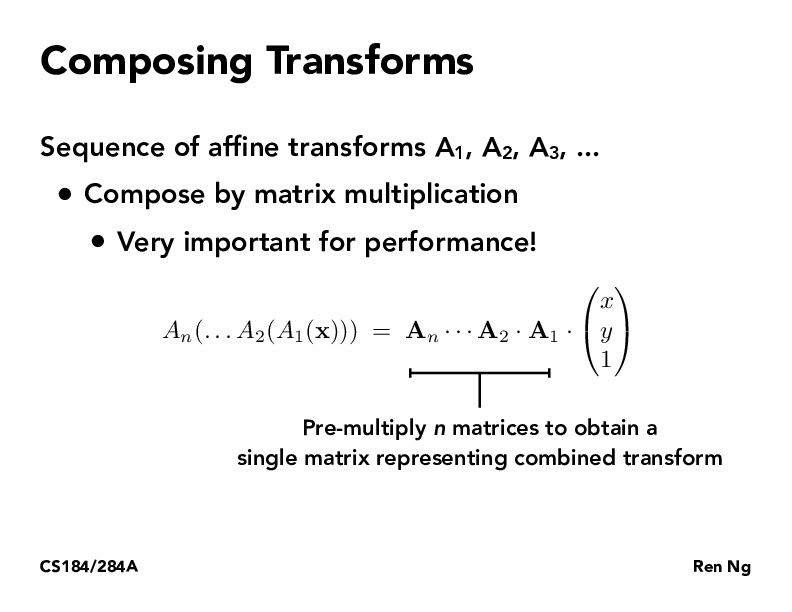Lecture 4: Transforms (34)Magicfulness

We can further optimize matrix multiplications by multiplying them in a specific order. We can find this order using Dynamic Programming, as described in CS170. More information: https://en.wikipedia.org/wiki/Matrix_chain_multiplicationandrewdotwang

Could this be optimized through the use of Fast Fourier Transform? Seems like this would help improve performance for matrix multiplications.katamarisun

Good question @Andrew; general matrices, unfortunately, do not have the nice recursive structure that the Fourier transform matrix does, thus explaining all the GPU and matrix acceleration efforts to speed up matrix multiplication.

You must be enrolled in the course to comment